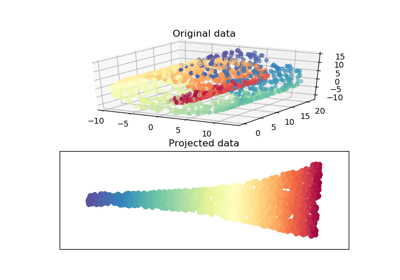/scikit-learn

# sklearn.manifold.locally_linear_embedding

`sklearn.manifold.locally_linear_embedding(X, n_neighbors, n_components, reg=0.001, eigen_solver=’auto’, tol=1e-06, max_iter=100, method=’standard’, hessian_tol=0.0001, modified_tol=1e-12, random_state=None, n_jobs=None)` [source]

Perform a Locally Linear Embedding analysis on the data.

Read more in the User Guide.

Parameters: `X : {array-like, NearestNeighbors}` Sample data, shape = (n_samples, n_features), in the form of a numpy array or a NearestNeighbors object. `n_neighbors : integer` number of neighbors to consider for each point. `n_components : integer` number of coordinates for the manifold. `reg : float` regularization constant, multiplies the trace of the local covariance matrix of the distances. `eigen_solver : string, {‘auto’, ‘arpack’, ‘dense’}` auto : algorithm will attempt to choose the best method for input data `arpack : use arnoldi iteration in shift-invert mode.` For this method, M may be a dense matrix, sparse matrix, or general linear operator. Warning: ARPACK can be unstable for some problems. It is best to try several random seeds in order to check results. `dense : use standard dense matrix operations for the eigenvalue` decomposition. For this method, M must be an array or matrix type. This method should be avoided for large problems. `tol : float, optional` Tolerance for ‘arpack’ method Not used if eigen_solver==’dense’. `max_iter : integer` maximum number of iterations for the arpack solver. `method : {‘standard’, ‘hessian’, ‘modified’, ‘ltsa’}` `standard : use the standard locally linear embedding algorithm.` see reference  `hessian : use the Hessian eigenmap method. This method requires` n_neighbors > n_components * (1 + (n_components + 1) / 2. see reference  `modified : use the modified locally linear embedding algorithm.` see reference  `ltsa : use local tangent space alignment algorithm` see reference  `hessian_tol : float, optional` Tolerance for Hessian eigenmapping method. Only used if method == ‘hessian’ `modified_tol : float, optional` Tolerance for modified LLE method. Only used if method == ‘modified’ `random_state : int, RandomState instance or None, optional (default=None)` If int, random_state is the seed used by the random number generator; If RandomState instance, random_state is the random number generator; If None, the random number generator is the RandomState instance used by `np.random`. Used when `solver` == ‘arpack’. `n_jobs : int or None, optional (default=None)` The number of parallel jobs to run for neighbors search. `None` means 1 unless in a `joblib.parallel_backend` context. `-1` means using all processors. See Glossary for more details. `Y : array-like, shape [n_samples, n_components]` Embedding vectors. `squared_error : float` Reconstruction error for the embedding vectors. Equivalent to `norm(Y - W Y, 'fro')**2`, where W are the reconstruction weights.

#### References

  (1, 2) `Roweis, S. & Saul, L. Nonlinear dimensionality reduction by locally linear embedding. Science 290:2323 (2000).`
  (1, 2) `Donoho, D. & Grimes, C. Hessian eigenmaps: Locally linear embedding techniques for high-dimensional data. Proc Natl Acad Sci U S A. 100:5591 (2003).`
  (1, 2) `Zhang, Z. & Wang, J. MLLE: Modified Locally Linear Embedding Using Multiple Weights.` http://citeseerx.ist.psu.edu/viewdoc/summary?doi=10.1.1.70.382
  (1, 2) `Zhang, Z. & Zha, H. Principal manifolds and nonlinear dimensionality reduction via tangent space alignment. Journal of Shanghai Univ. 8:406 (2004)`

## Examples using `sklearn.manifold.locally_linear_embedding`Swiss Roll reduction with LLE